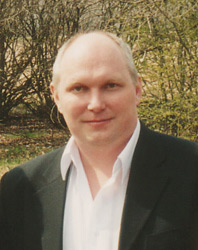Zero Velocity Photon
by Alexander V. Frolov

This research project is proposed to investigate some possible ways for creation of different kind of longitudinal waves of energy  density. The notion of longitudinal electromagnetic wave is described here is original author's notion.

In general sense a "longitudinal wave" is oscillations of energy density that is produced in the direction of the wave movement. The example is acoustical wave in air that is alternating sinusoidal compression and decompression of air. Energy density here is described as potential energy of molecular interaction for areas of different density of air, i.e. for different distances between molecules.

Electromagnetic wave by Hertz is not longitudinal if E (electric intensity vector) and H (magnetic intensity vector) are considered as energy density parameters. But it is not true understanding of the nature for this wave since the energy density is described here by Poynting vector P (that is known in Russia  also as Umov vector) and this vector direction is perpendicular both to E and H. The direction of P for Hertz electromagnetic wave and direction of its movement is the same. In other words, the reason for motion of photon is energy translation in Poynting vector direction and the wave is longitudinal one.

From this new understanding there are several important technological conclusions:

1. The velocity of photon can be increased or decreased since it is depend of vector P value.
2. Poynting vector for Hertz electromagnetic wave is uni-directional pulse function and by this description this kind of wave should be named as pulsing longitudinal wave.
3. Classical longitudinal wave of sinusoidal type (acoustic wave) can be created by some electromagnetic source for sinusoidal changes of Poynting vector value and direction. There are no condition for movement of the wave in this case since it is oscillating in both directions. The name for it is proposed here as zero velocity photon (ZVP).

I suppose this zero velocity photons (ZVP) are known "sea of hidden energy" or zero-point energy (ZPE) that is described by many authors. But just now the difference between moving photon and zero-point photon is disclosed: it is parameters of vector Poynting function P(t). For moving photon it is uni-directional pulses and for ZPE photons it is alternating sinusoidal oscillations.

Zero velocity photons are source for known case of electron-positron materialization. In resonance interaction with space there is technical possibility for stable process of matter production or matter decomposition. It is the base for teleportation as a new kind of transport technologies.

Force interaction (pressure of the wave) is known from Hertz wave properties. In special kind wave it is possible to create attraction force if the pulses of Poynting vector are asymmetrical. Energy density waves are moving to source in this case but not from the source. In some theoretical papers it was described as "black beam" process.

There are number of technical methods for longitudinal wave generation. N.Tesla worked on high voltage and high frequency source for some constant size of the capacitor surface. It is proposed here to use changes of charged surface area when the potential value is constant. The method is described by K.P.Butusov  St.Petersburg. Technically it is more simple to work with cylinder of changing volume instead of oscillating volume sphere. High frequency waves of optic range can be produced by special optic equipment with non-linear elements to get the possibility for modulation by vector Poynting value and direction.

Let's note: The density is notion for some certain type of space: for line, surface or volume. Density of electric current is an example of linear density of energy. In special case it is possible to design the system of four dimensional energy density oscillations. The result for this wave is energy transference in time axis direction. Theoretically it is possible in both directions: energy can be moving in Present State of some system from Past State of the system or it can be incoming from Future State of the system. Four dimensional transference should be described by four dimensional vector that is analogy with known Poynting vector.

Practical applications for this technology are gravity and time rate control, since velocity of photon and rate of time are correlated values in Kozyrev's conception. The waves can not be screened and it can be used for new kind telecommunication. There are some medical aspects also.

The technology allow to create reactionless propulsion for space-craft and it was proved by Academician Ignatyev's research.

The conception disclosed here allow us to suppose elastic properties of Ether, so energy density waves in Ether should be considered by analogy with air density waves. In this case energy of local area of space is function of Ether density, i.e. function of distance between its elements. There are clear analogy here with N.Kozyrev's notion for "density of time flow". Some technologies for time rate control were proposed those are based on controlled changes of this density. There are no needs in relativistic velocities of motion to get the time-travel in this conception. The Time is property of reality and it can be controlled by space-time engineering technologies.

Copyright 1999 Alexander V. Frolov. All right reserved.a2509@yahoo.com